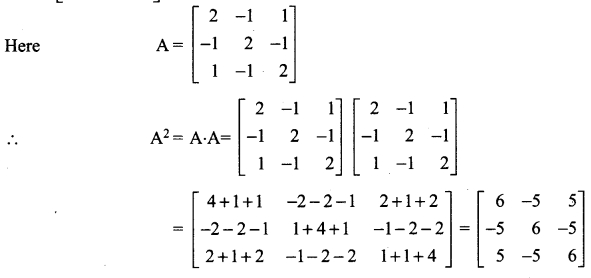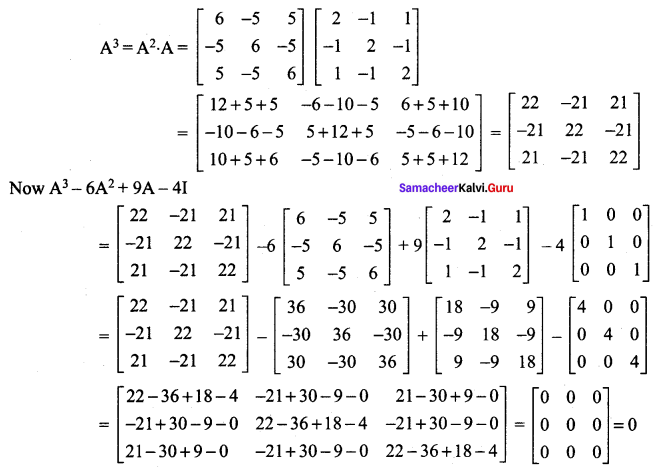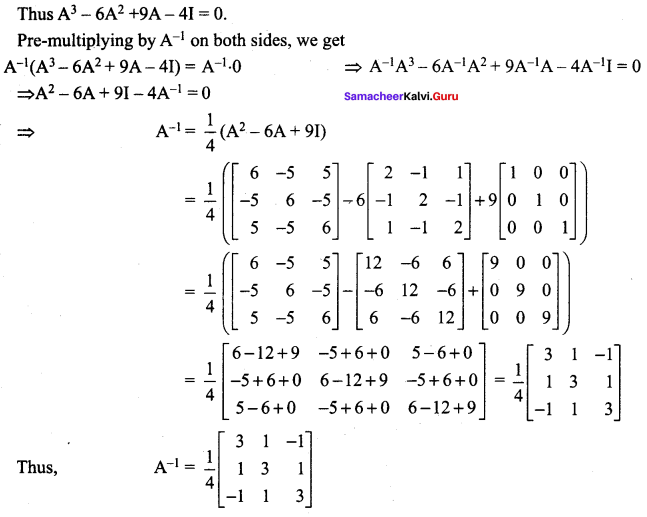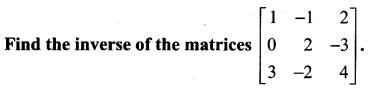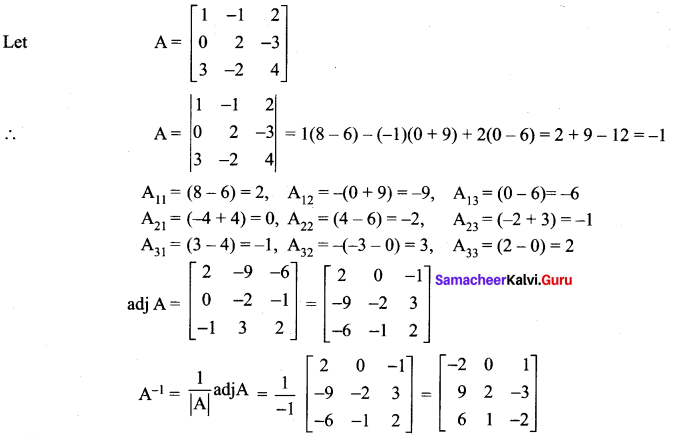# Samacheer Kalvi 12th Maths Solutions Chapter 1 Applications of Matrices and Determinants Ex 1.1

## Tamilnadu Samacheer Kalvi 12th Maths Solutions Chapter 1 Applications of Matrices and Determinants Ex 1.1

Exercise 1.1 Class 12 Maths State Board Question 1.
Find the adjoint of the following: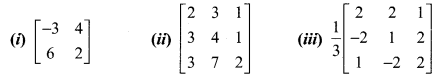Solution: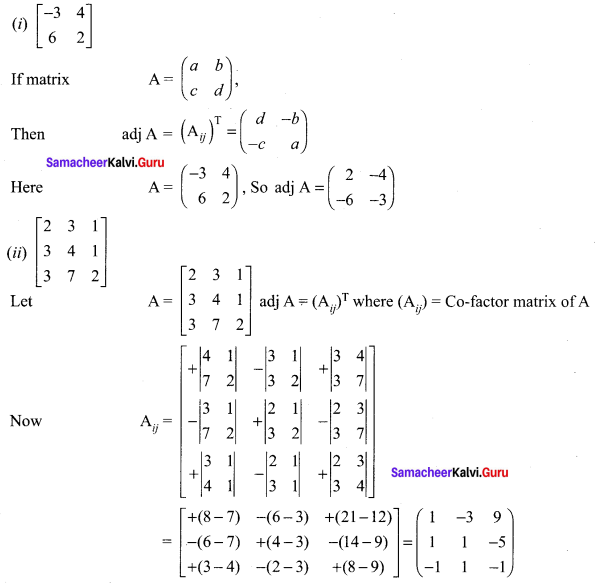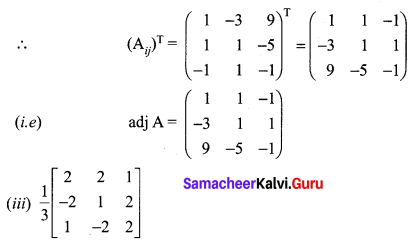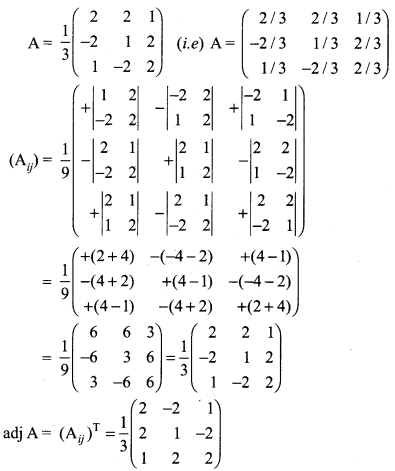12th Maths Exercise 1.1 Answers Question 2.
Find the inverse (if it exists) of the following: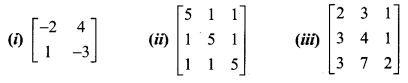Solution:
For a matrix A, $$\mathrm{A}^{-1}=\frac{1}{|\mathrm{A}|}(\mathrm{adj} \mathrm{A})$$. Where |A| ≠ 0. If |A| = 0 then A is called a singular matrix and so $$\mathrm{A}^{-1}$$ does not exist.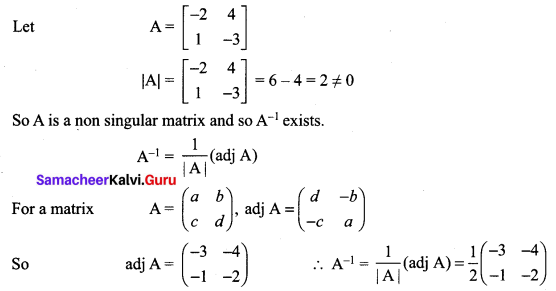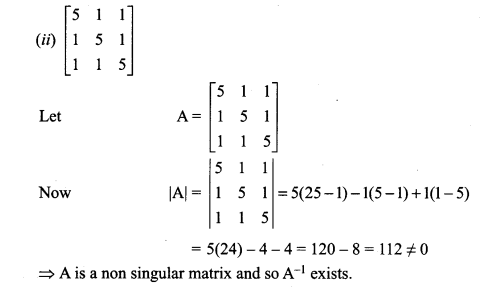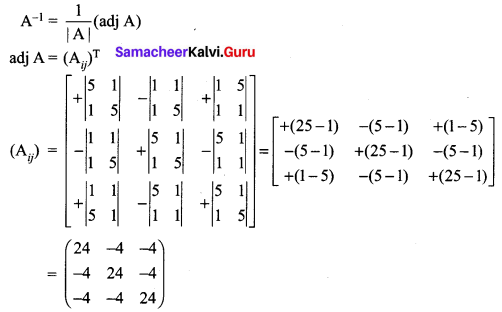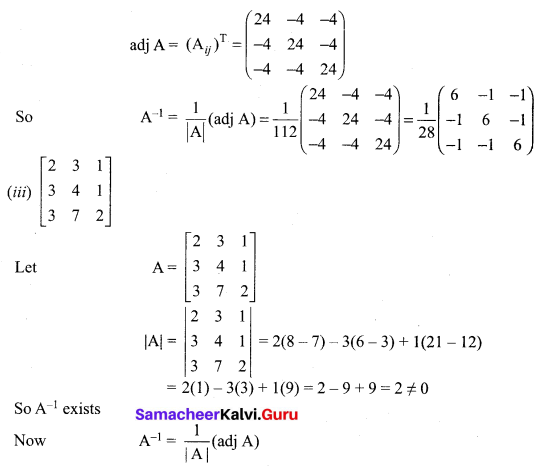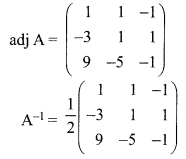12th Maths Exercise 1.1 Question 3.
If F(α) = $$\left[\begin{array}{ccc}{\cos \alpha} & {0} & {\sin \alpha} \\ {0} & {1} & {0} \\ {-\sin \alpha} & {0} & {\cos \alpha}\end{array}\right]$$ show that $$[\mathrm{F}(\alpha)]^{-1}=\mathrm{F}(-\alpha)$$
Solution:
Let A = F (α)
So $$[\mathrm{F}(\alpha)]^{-1}=\mathrm{A}^{-1}$$
Now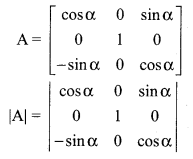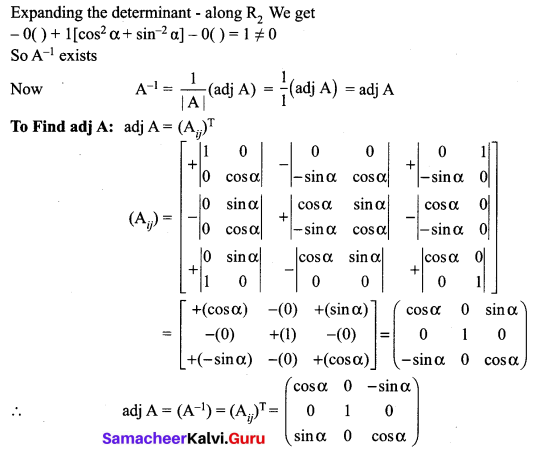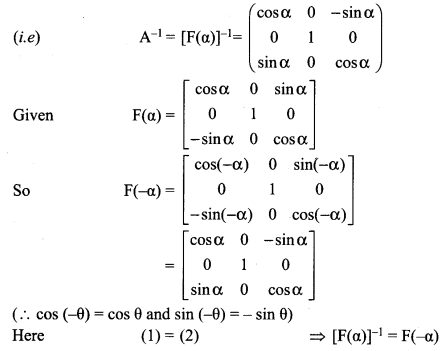12th Maths Chapter 1 Exercise 1.1 Question 4.
If A = $$\left[\begin{array}{cc}{5} & {3} \\ {-1} & {-2}\end{array}\right]$$ show that A2 – 3A – 7I2 = O2. Hence find A-1.
Solution:
A = $$\left[\begin{array}{cc}{5} & {3} \\ {-1} & {-2}\end{array}\right]$$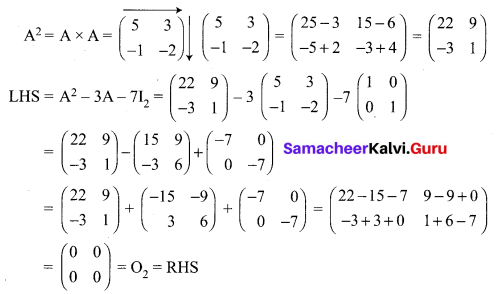To Find A-1
Now we have proved that A2 – 3A – 7I2 = O2
Post multiply by A-1 we get
A – 3I – 7A-1 = O2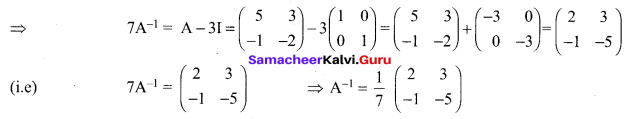If $$\mathbf{A}=\frac{1}{9}\left[\begin{array}{ccc}{-8} & {1} & {4} \\ {4} & {4} & {7} \\ {1} & {-8} & {4}\end{array}\right]$$ prove that A-1 = AT
Solution: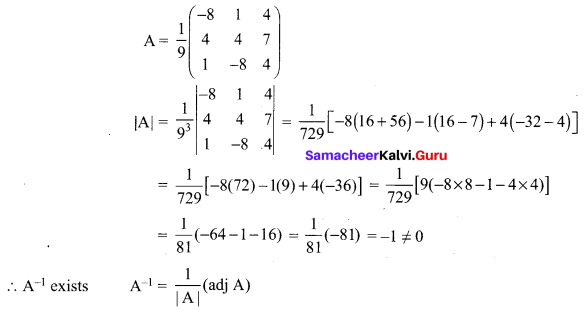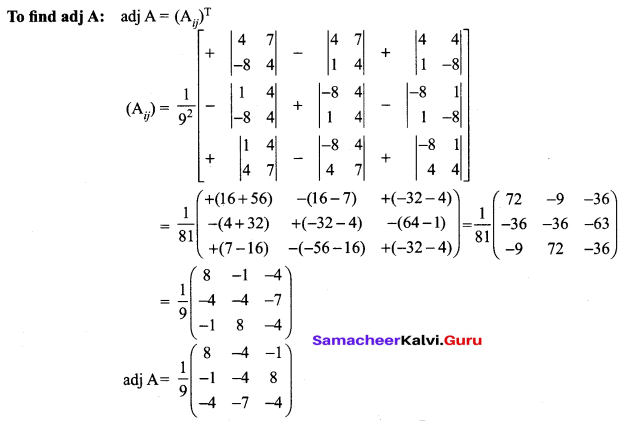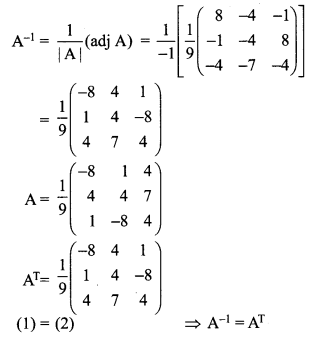If $$\mathbf{A}=\left[\begin{array}{rr}{8} & {-4} \\ {-5} & {3}\end{array}\right]$$, verify that A(adj A) = (adj A)A = |A| I2
Solution: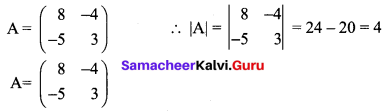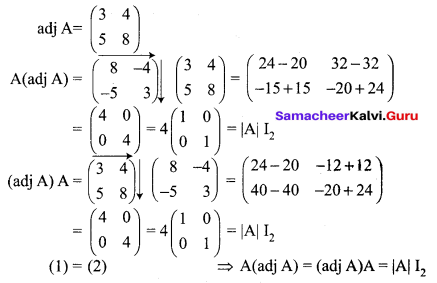Samacheer Kalvi 12 Maths Solutions Question 7.
If $$\mathbf{A}=\left[\begin{array}{ll}{3} & {2} \\ {7} & {5}\end{array}\right]$$, and $$\mathbf{B}=\left[\begin{array}{cc}{-1} & {-3} \\ {5} & {2}\end{array}\right]$$ verify that (AB)-1 = B-1 A-1.
Solution: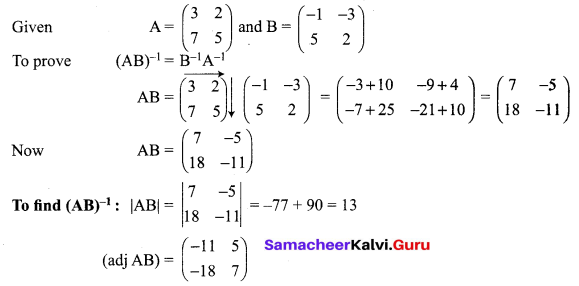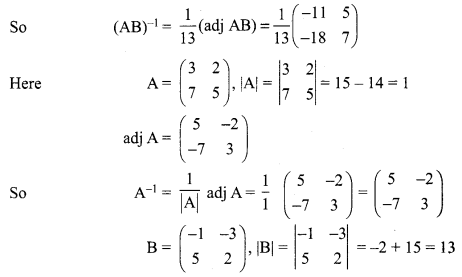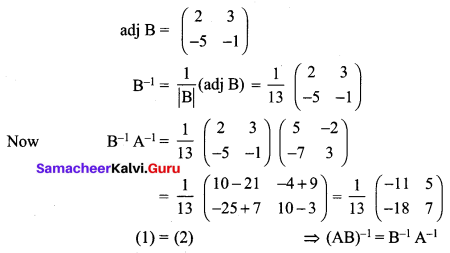Samacheer Kalvi Guru 12th Maths Question 8.
If adj (A) = $$\left[\begin{array}{ccc}{2} & {-4} & {2} \\ {-3} & {12} & {-7} \\ {-2} & {0} & {2}\end{array}\right]$$ find A
Solution: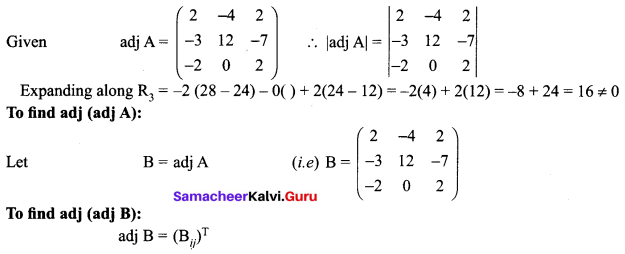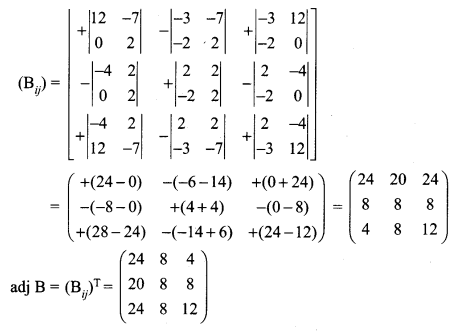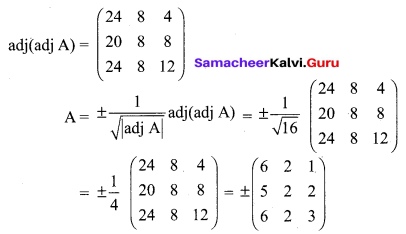If adj(A) = $$\left[\begin{array}{ccc}{0} & {-2} & {0} \\ {6} & {2} & {-6} \\ {-3} & {0} & {6}\end{array}\right]$$ find A-1
Solution: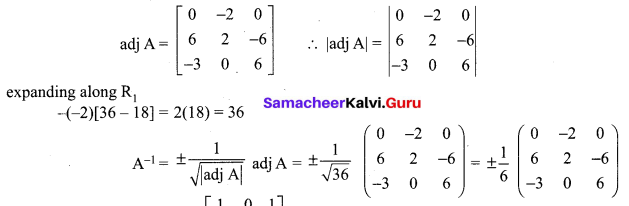Samacheer Kalvi 12th Maths Guide Question 10.
Find adj(adj(A)) if adj A = $$\left[\begin{array}{ccc}{1} & {0} & {1} \\ {0} & {2} & {0} \\ {-1} & {0} & {1}\end{array}\right]$$
Solution: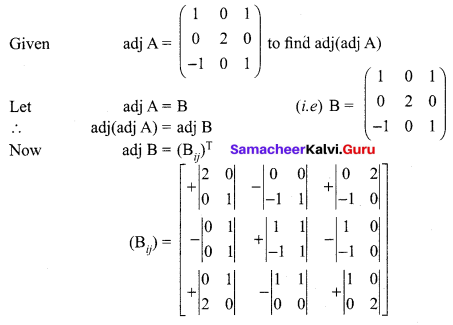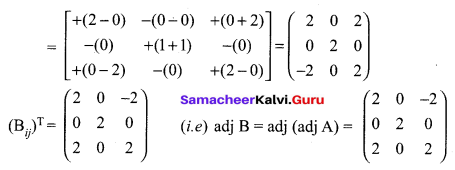12th Samacheer Maths Solutions Question 11.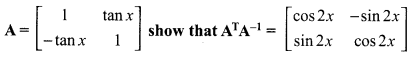Solution: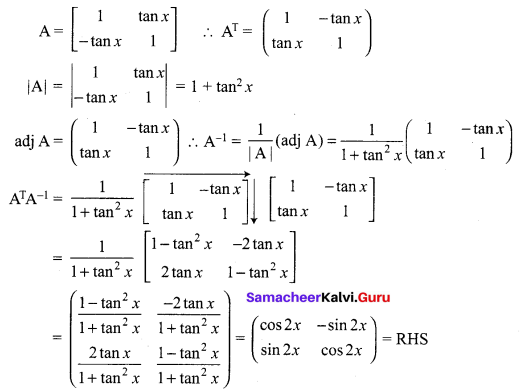12th Maths 1st Chapter Exercise 1.1 Question 12.
Find the matrix A for which A $$\left[\begin{array}{cc}{5} & {3} \\ {-1} & {-2}\end{array}\right]=\left[\begin{array}{cc}{14} & {7} \\ {7} & {7}\end{array}\right]$$
Solution:
Given A $$\left[\begin{array}{cc}{5} & {3} \\ {-1} & {-2}\end{array}\right]=\left[\begin{array}{cc}{14} & {7} \\ {7} & {7}\end{array}\right]$$
Let $$\mathrm{B}=\left(\begin{array}{cc}{5} & {3} \\ {-1} & {-2}\end{array}\right) \text { and } \mathrm{C}=\left(\begin{array}{cc}{14} & {7} \\ {7} & {7}\end{array}\right)$$
Given AB = C, To find A
Now AB = C
Post multiply by B-1 on both sides
ABB-1 = CB-1 (i.e) A (BB-1) = CB-1
⇒ A(I) = CB-1 (i.e) A = CB-1
To find B-1: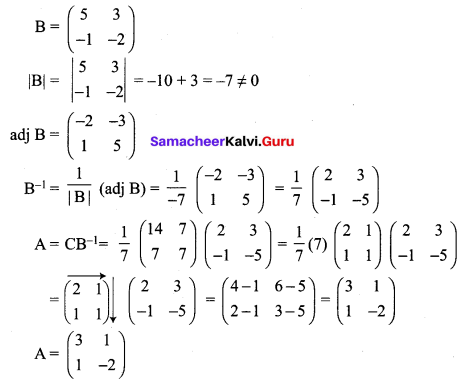12th Maths Solution Book Question 13.
Given $$\mathbf{A}=\left[\begin{array}{cc}{1} & {-1} \\ {2} & {0}\end{array}\right], \mathbf{B}=\left[\begin{array}{cc}{3} & {-2} \\ {1} & {1}\end{array}\right] \text { and } \mathbf{C}\left[\begin{array}{ll}{1} & {1} \\ {2} & {2}\end{array}\right]$$, find a matrix X such that AXB = C.
Solution:
A × B = C
Pre multiply by A-1 and post multiply by B-1 we get
A-1 A × BB-1 = A-1CB-1 (i.e) X = A-1CB-1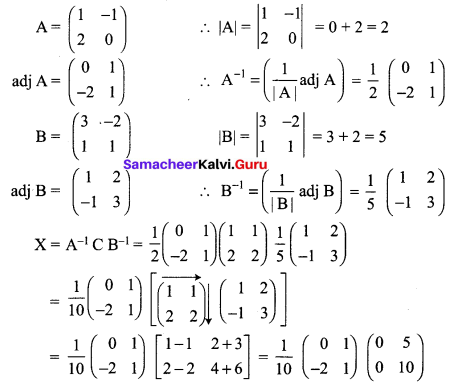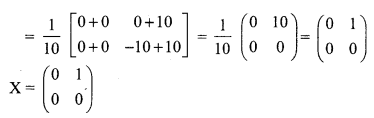Maths Guide For Class 12 Question 14.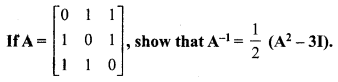Solution: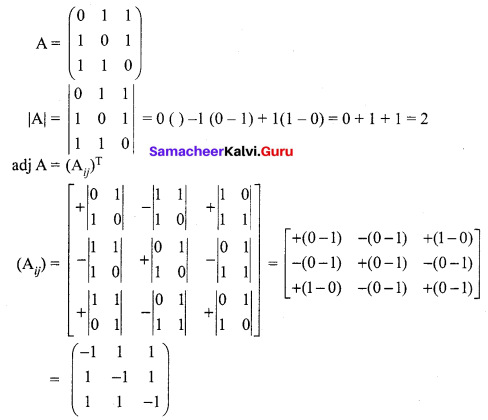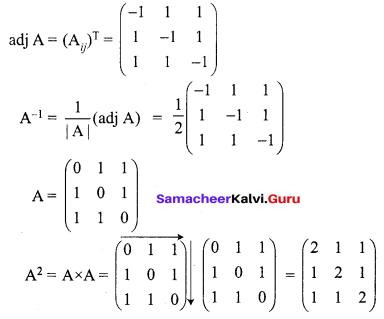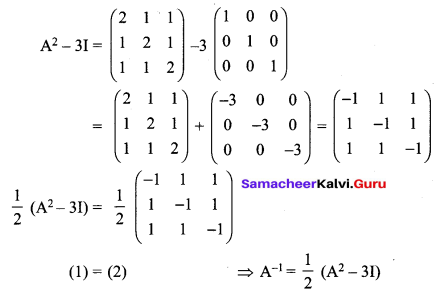Maths 12th Guide Question 15.
Decrypt the received encoded message $$\left[\begin{array}{cc}{2} & {-3}\end{array}\right]\left[\begin{array}{ll}{20} & {4}\end{array}\right]$$ with the encryption matrix $$\left[\begin{array}{cc}{-1} & {-1} \\ {2} & {1}\end{array}\right]$$ and the decryption matrix as its inverse, where the system of codes are described by the numbers 1-26 to the letters A- Z respectively, and the number 0 to a blank space.
Solution:
Let the encoding matrix be $$\left[\begin{array}{cc}{-1} & {-1} \\ {2} & {1}\end{array}\right]$$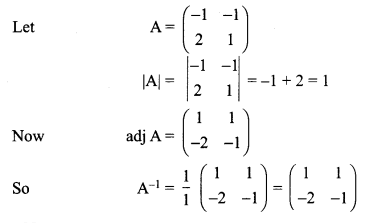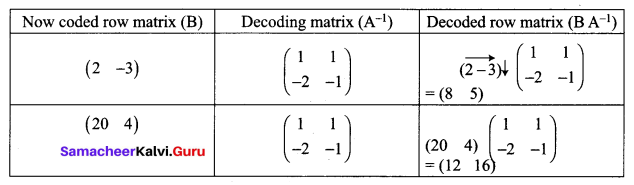So the sequence of decoded matrices is [8 5], [12 16].

### Samacheer Kalvi 12th Maths Solutions Chapter 1 Applications of Matrices and Determinants Ex 1.1 Additional Problems

Using elementary transformations find the inverse of the following matrix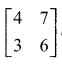Solution: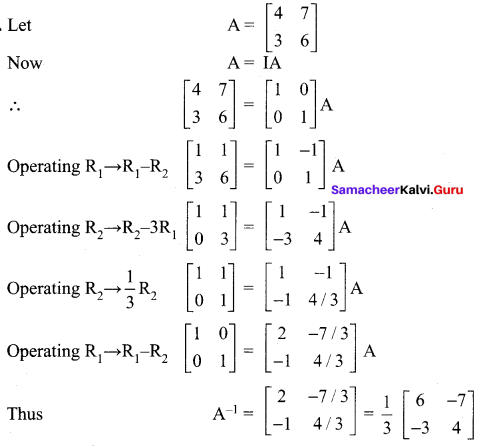Using elementary transformations find the inverse of the matrix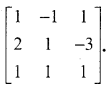Solution: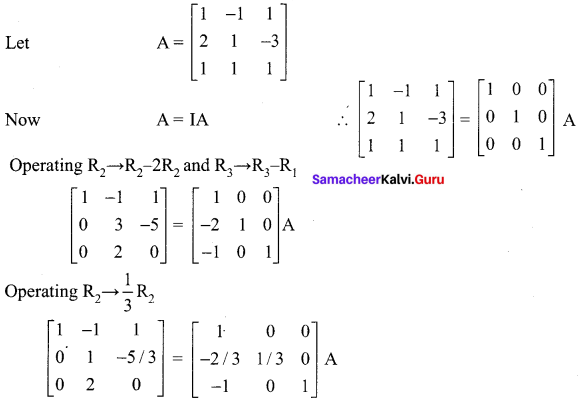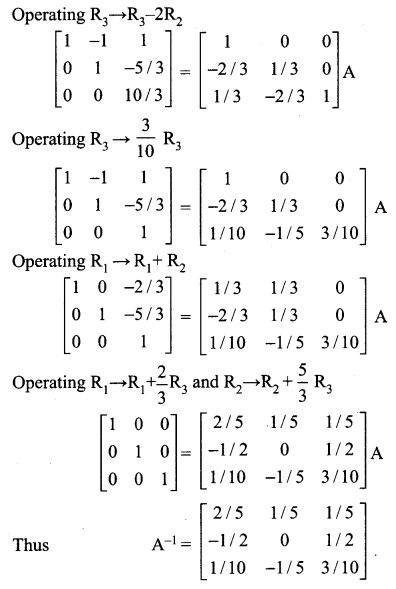12th Maths Guide Samacheer Kalvi Question 3.
Using elementary transformation find the inverse of the matrix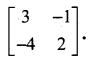Solution: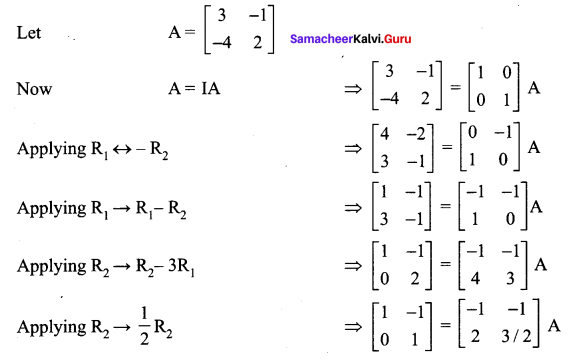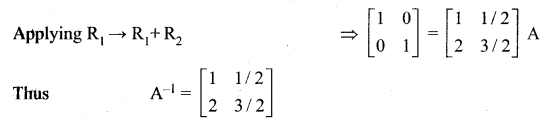12th Maths Book Back Answers Question 4.
Using elementary transformations find the inverse of the matrix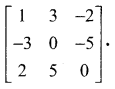Solution: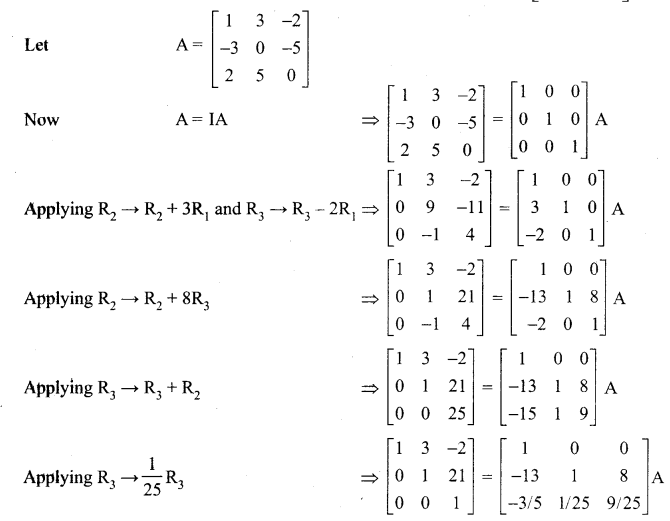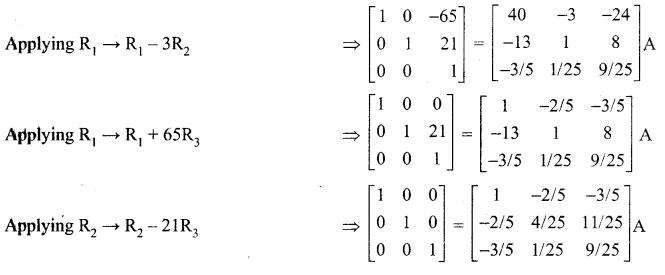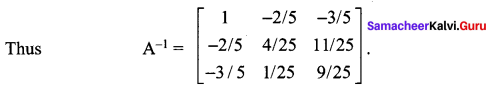12th Maths Samacheer Solutions Question 5.
Using elementary transformation, find the inverse of the following matrix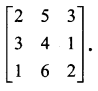Solution: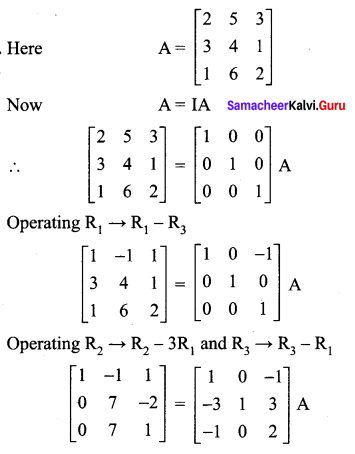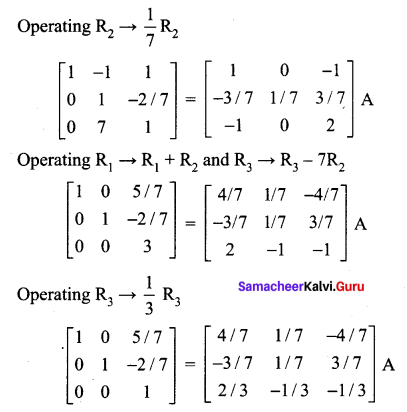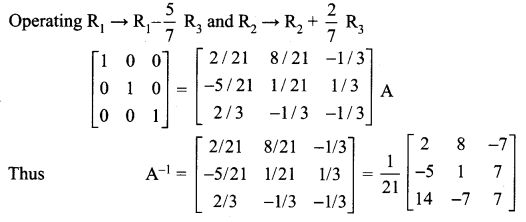12th Samacheer Maths Solution Question 6.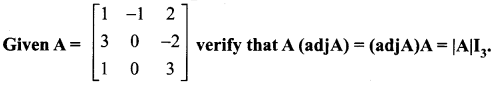Solution: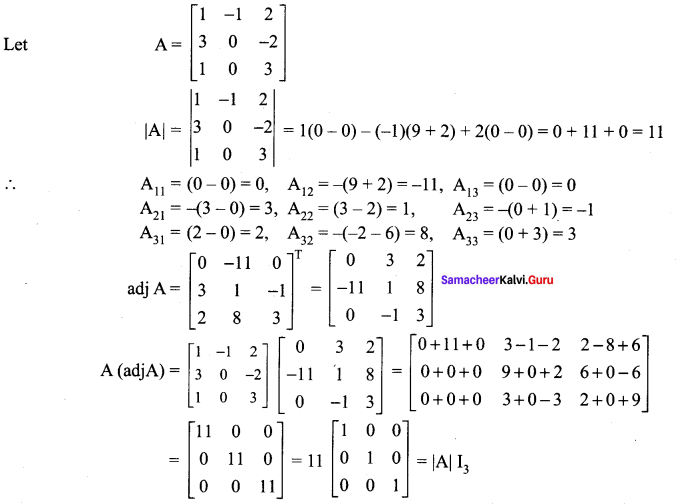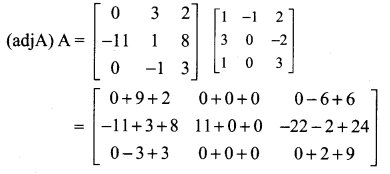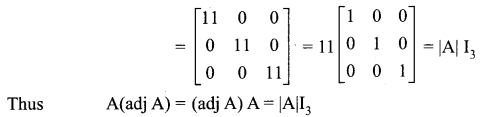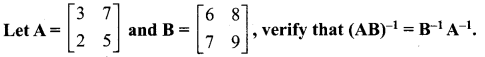Solution: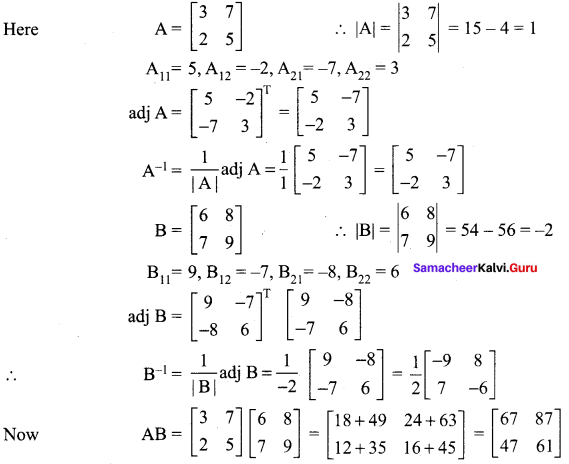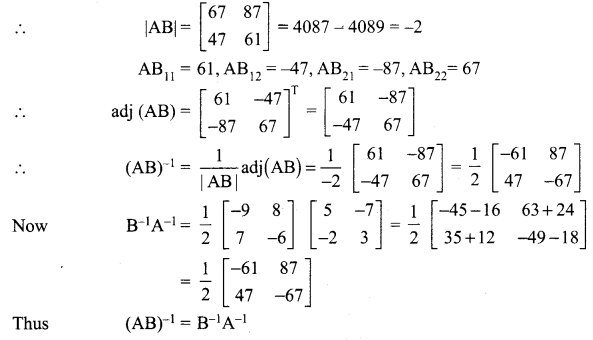12th Maths Book Back Questions With Answers Question 8.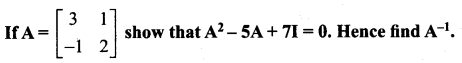Solution: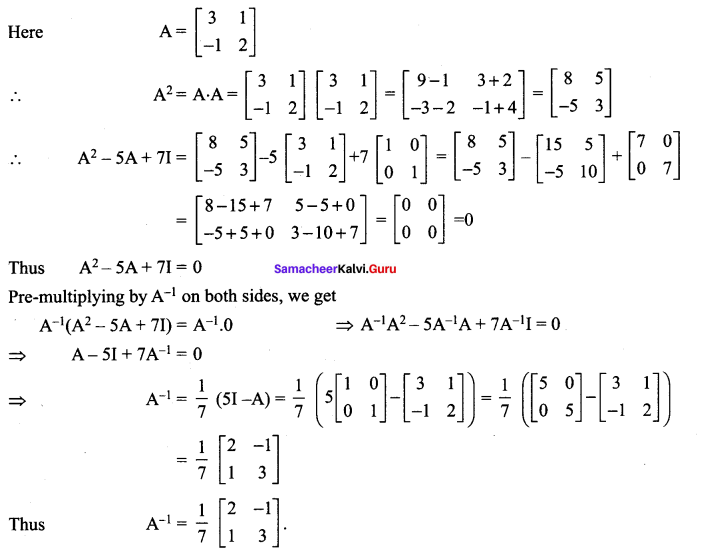12th Samacheer Maths Guide Question 9.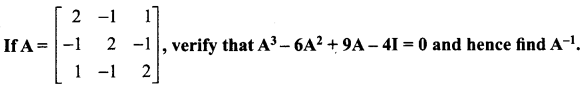Solution: